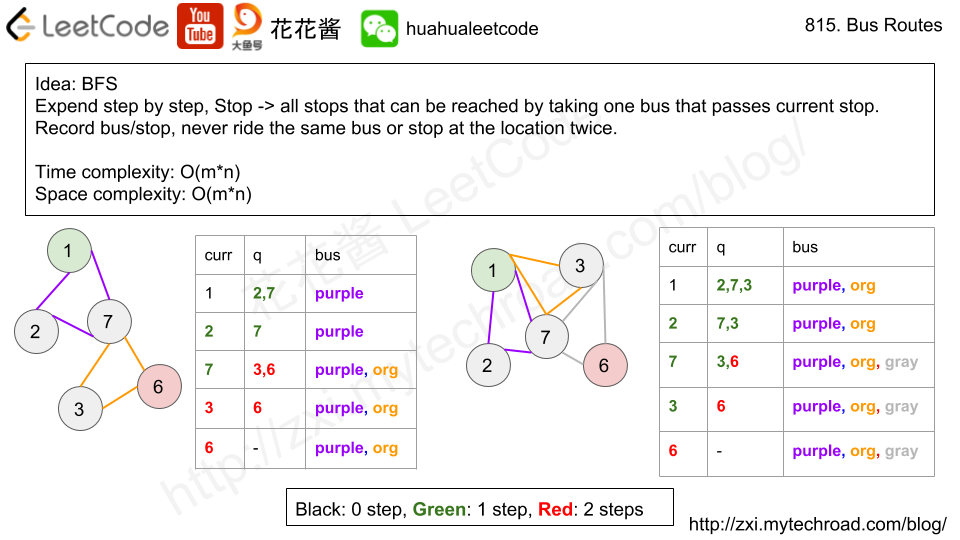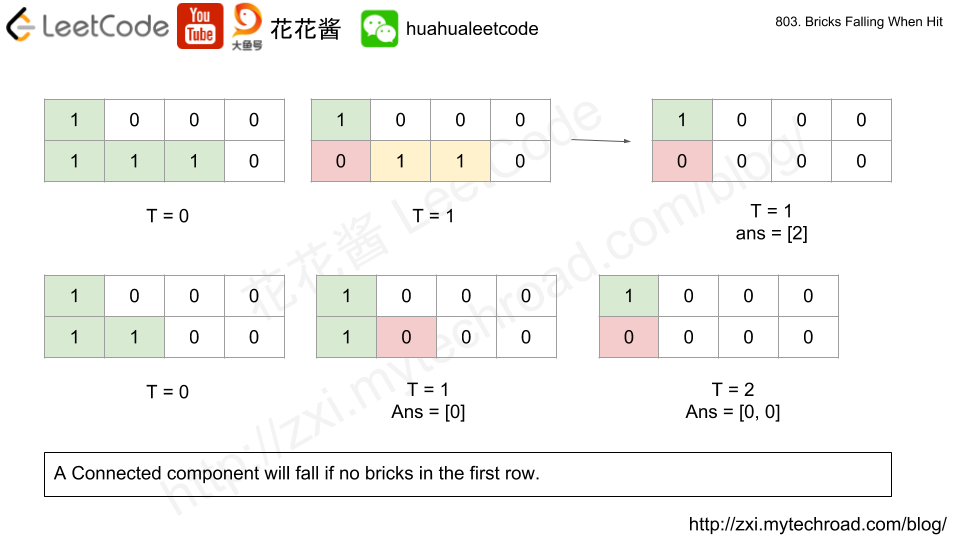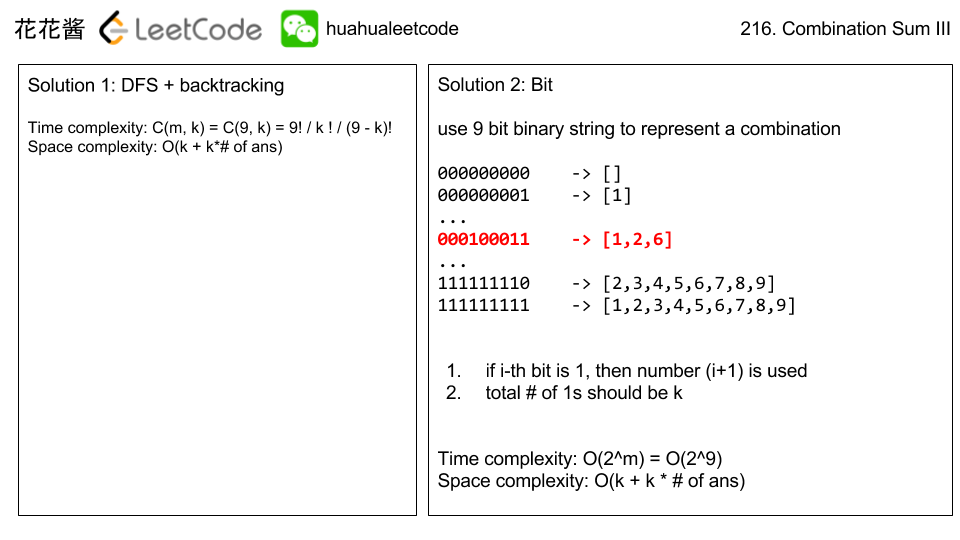# Problem

https://leetcode.com/problems/bus-routes/description/

We have a list of bus routes. Each routes[i] is a bus route that the i-th bus repeats forever. For example if routes = [1, 5, 7], this means that the first bus (0-th indexed) travels in the sequence 1->5->7->1->5->7->1->… forever.

We start at bus stop S (initially not on a bus), and we want to go to bus stop T. Travelling by buses only, what is the least number of buses we must take to reach our destination? Return -1 if it is not possible.

Example:
Input:
routes = [[1, 2, 7], [3, 6, 7]]
S = 1
T = 6
Output: 2
Explanation:
The best strategy is take the first bus to the bus stop 7, then take the second bus to the bus stop 6.


Note:

• 1 <= routes.length <= 500.
• 1 <= routes[i].length <= 500.
• 0 <= routes[i][j] < 10 ^ 6.# Solution: BFS

Time Complexity: O(m*n) m: # of buses, n: # of routes

Space complexity: O(m*n + m)

C++

# Problem

In a given integer array A, we must move every element of A to either list B or list C. (B and C initially start empty.)

Return true if and only if after such a move, it is possible that the average value of B is equal to the average value of C, and B and C are both non-empty.

Example :
Input:
[1,2,3,4,5,6,7,8]
Output: true
Explanation: We can split the array into [1,4,5,8] and [2,3,6,7], and both of them have the average of 4.5.


Note:

• The length of A will be in the range [1, 30].
• A[i] will be in the range of [0, 10000].

# Solution: Search

Time complexity: O(2^n)

Space complexity: O(n)

# Problem

We have a grid of 1s and 0s; the 1s in a cell represent bricks.  A brick will not drop if and only if it is directly connected to the top of the grid, or at least one of its (4-way) adjacent bricks will not drop.

We will do some erasures sequentially. Each time we want to do the erasure at the location (i, j), the brick (if it exists) on that location will disappear, and then some other bricks may drop because of that erasure.

Return an array representing the number of bricks that will drop after each erasure in sequence.

Example 1:
Input:
grid = [[1,0,0,0],[1,1,1,0]]
hits = [[1,0]]
Output: 
Explanation:
If we erase the brick at (1, 0), the brick at (1, 1) and (1, 2) will drop. So we should return 2.
Example 2:
Input:
grid = [[1,0,0,0],[1,1,0,0]]
hits = [[1,1],[1,0]]
Output: [0,0]
Explanation:
When we erase the brick at (1, 0), the brick at (1, 1) has already disappeared due to the last move. So each erasure will cause no bricks dropping.  Note that the erased brick (1, 0) will not be counted as a dropped brick.

# Idea

1. For each day, hit and clear the specified brick.
2. Find all connected components (CCs) using DFS.
3. For each CC, if there is no brick that is on the first row that the entire cc will drop. Clear those CCs.# Related Problems

Problem:

Given a digit string, return all possible letter combinations that the number could represent.

A mapping of digit to letters (just like on the telephone buttons) is given below.Note:
Although the above answer is in lexicographical order, your answer could be in any order you want.

Solution 1: DFS

Solution 2:  BFS

## Python

Problem:

Find all possible combinations of k numbers that add up to a number n, given that only numbers from 1 to 9 can be used and each combination should be a unique set of numbers.

Example 1:

Input: k = 3, n = 7

Output:

Example 2:

Input: k = 3, n = 9

Output:

Idea:

DFS + backtracking

bitSolution:

C++

C++ / binary

Python

Related problems:

Mission News Theme by Compete Themes.